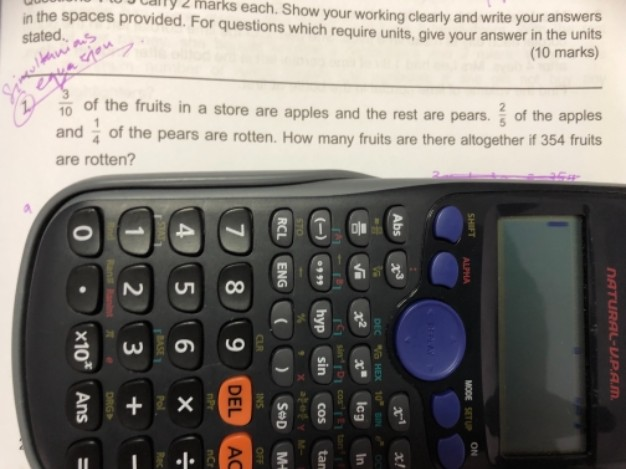# QuestionAssuming total fruit is 10 unit, 3 unit is apple, 7 unit is pears

3 unit x 2/5 = 6/5 unit rotten apple

7 unit x 1/4 = 7/4 unit rotten pear

6/5 unit + 7/4 unit = 59/20 unit

59/20 unit = 354

1 unit = 354 x 20/59 = 120

120 x 10 = 1200

Ans: Total number is fruits is 1200

0 Replies 0 Likes